Question

Find the transfer function Given below is a block diagram of a load frequency control system...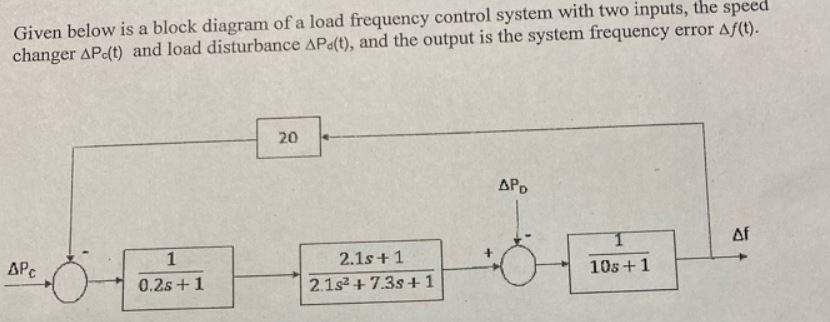Find the transfer function

Given below is a block diagram of a load frequency control system with two inputs, the speed changer AP.(t) and load disturbance Apa(t), and the output is the system frequency error Af(t). 20 APD APC 0.25 +1 2.1s +1 2.1s2 + 7.3s +1 10s +1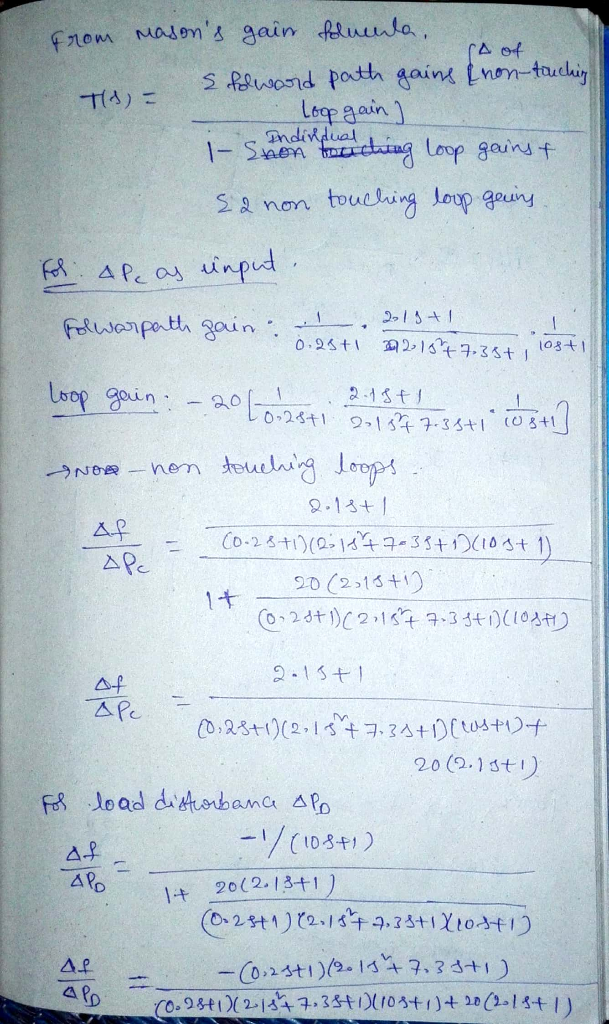Earn Coins

Coins can be redeemed for fabulous gifts.

Similar Homework Help Questions
• Motor Position Control with Torque Disturbance The following diagram models a motor position control system with...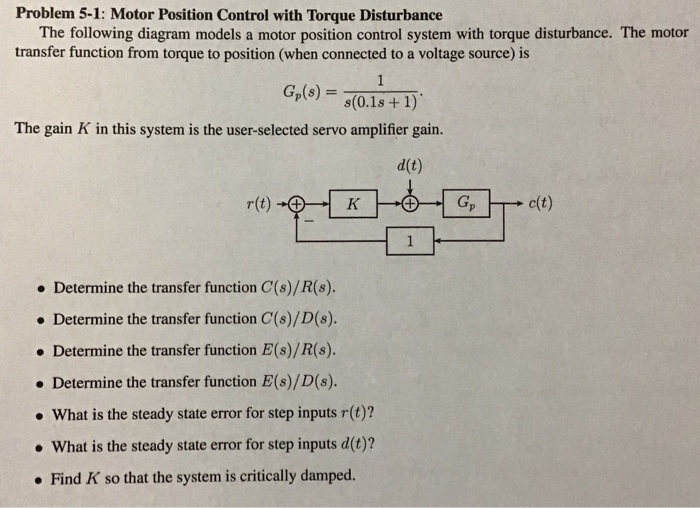Motor Position Control with Torque Disturbance The following diagram models a motor position control system with torque disturbance. The motor transfer function from torque to position (when connected to a voltage source) is G(s) = 1/s(0.1s + 1). Problem 5-1: Motor Position Control with Torque Disturbance The following diagram models a motor position control system with torque disturbance. The motor transfer function from torque to position (when connected to a voltage source) is s(0.1s +1) The gain K in this...

• P4: The car model of a cruise control system is given in the following transfer function...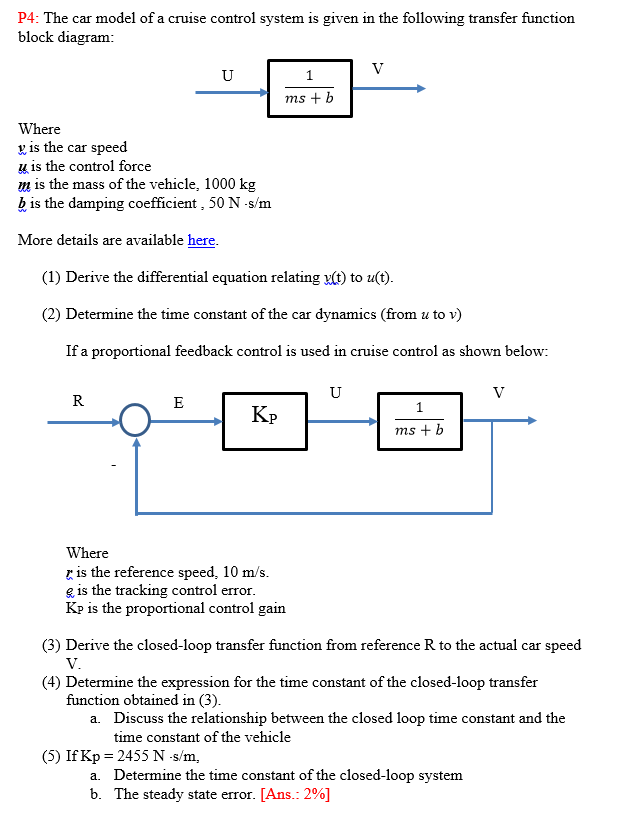P4: The car model of a cruise control system is given in the following transfer function block diagram ms + b Where v is the car speed u is the control force m is the mass of the vehicle, 1000 kg b is the damping coefficient, 50 N s/m More details are available here (1) Derive the differential equation relating y(t) to u(t) (2) Determine the time constant of the car dynamics (from u to v) If a proportional feedback...

• 4 (a) Based on the block diagram for a control system (Figure Q4(a) below) determine the...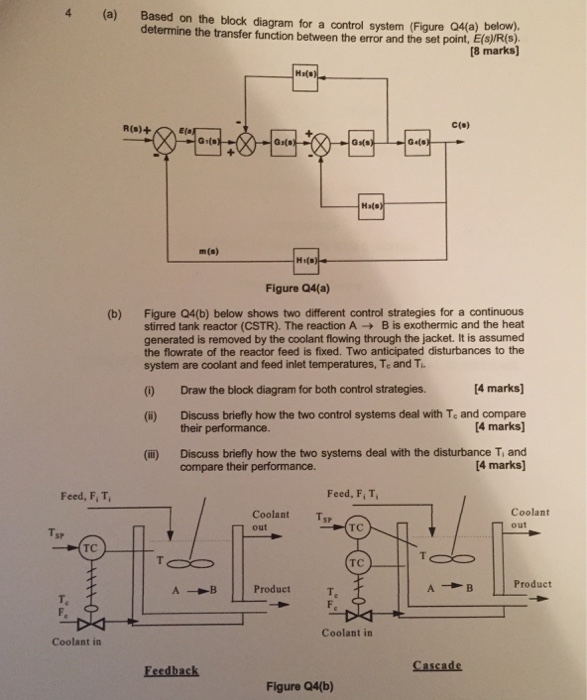4 (a) Based on the block diagram for a control system (Figure Q4(a) below) determine the transfer function between the error and the set point, E(s)/R(s) (8 marks) R(s)+ c(p) m(s) Figure Q4(a) Figure Q4(b) below shows two different control strategies for a continuous stirred tank reactor (CSTR). The reaction A → B is exothermic and the heat generated is removed by the coolant flowing through the jacket. It is assumed the flowrate of the reactor feed is fixed. Two...

• Question) The transfer function of the system given in the block diagram below a) Find with...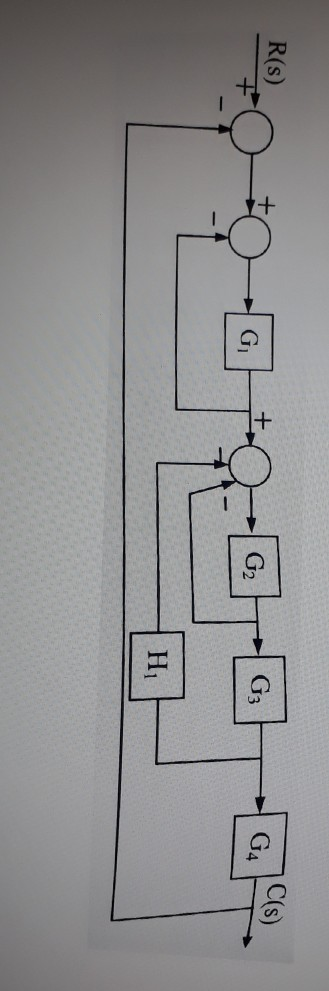Question) The transfer function of the system given in the block diagram below a) Find with the block diagram reduction method? b) Find by Routh-Hurwitz stability analysis method? NOTE: Draw option b on the flow diagram and solve it.    R(S) C(s) GA G3 G H

• 2. A feedback control system is subject to disturbances at the actuator input, as shown in the following block diagram. Remember that you need to use the final value theorem (and not the table) when...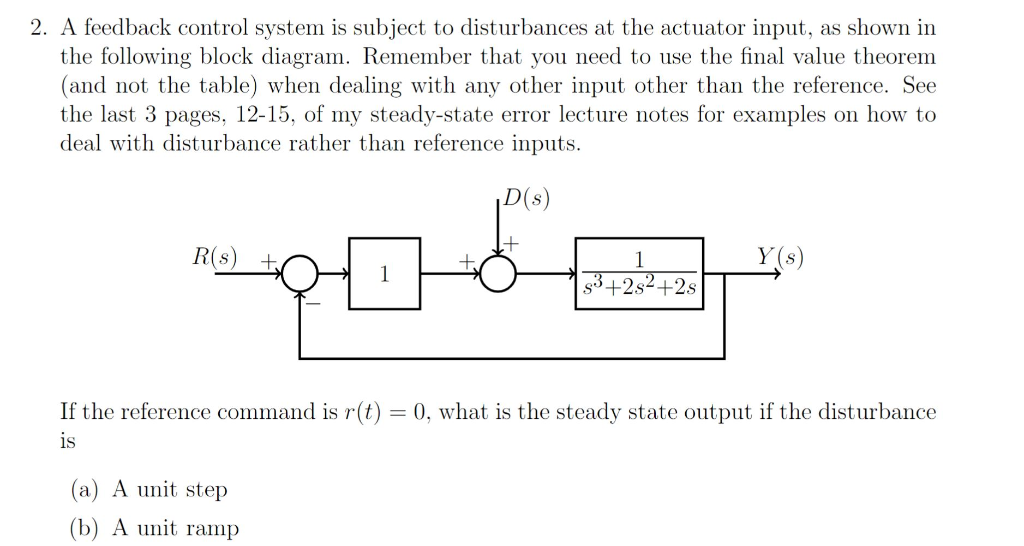2. A feedback control system is subject to disturbances at the actuator input, as shown in the following block diagram. Remember that you need to use the final value theorem (and not the table) when dealing with any other input other than the reference. See the last 3 pages, 12-15, of my steady-state error lecture notes for examples on how to deal with disturbance rather than reference inputs D(s) 1 Y(s) \$3+2s2+2s If the reference command is r(t) 1S 0,...

• Consider the block diagram of the following control system. Find the transfer function G(s) = Y(S)/R(s)...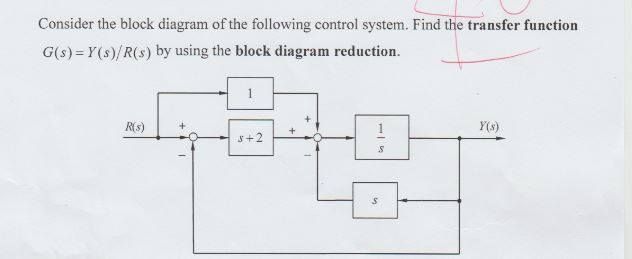Consider the block diagram of the following control system. Find the transfer function G(s) = Y(S)/R(s) by using the block diagram reduction R(5) Y(s) + 5+2 s

• The figure below shows a block diagram of a feedback system. Both cascade clutch and forward...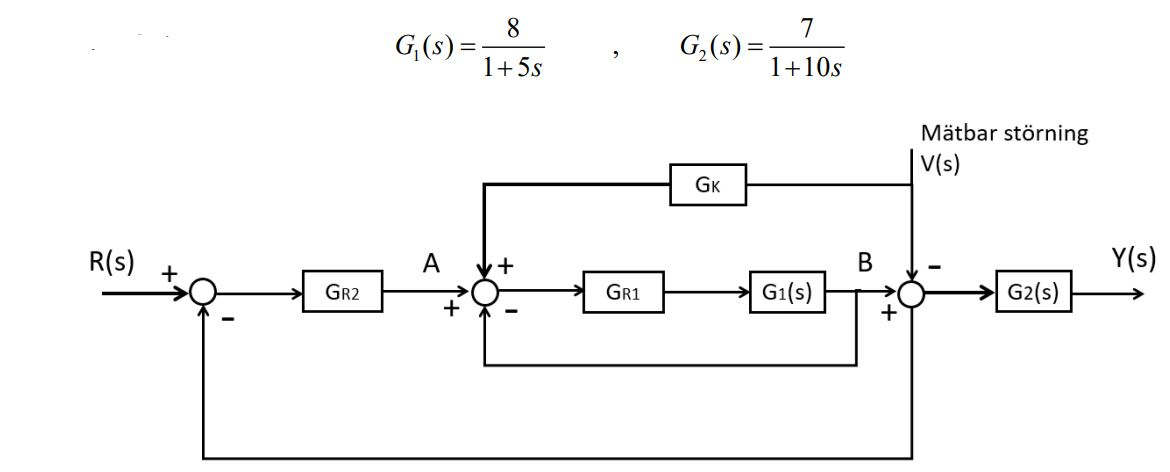The figure below shows a block diagram of a feedback system. Both cascade clutch and forward clutch are used to obtain the best possible control. G1 and G2 have the following transfer functions: G1(s) = 8 / 1+5s , G2(s)= 7/ 1+10s a) Suppose that a (t) = σ (t) or A (s) = 1 / s. Dimension Gr1 = K so that the residual error s e in the inner loop from A (s) to B (s) becomes less...

• Consider the liquid level control system given in the figure. The values with overbar denote stea...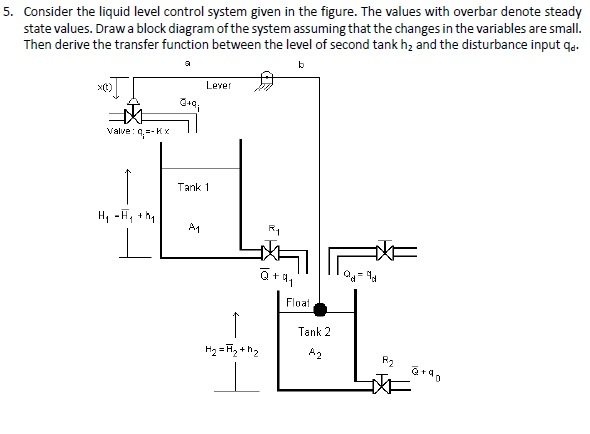Consider the liquid level control system given in the figure. The values with overbar denote steady state values. Draw a block diagram of the system assuming that the changes in the variables are small. Then derive the transfer function between the level of second tank h2 and the disturbance input qd 5. x(t) Lever Valve: q.-Kx Tank 1 O+q oa Tank 2 Consider the liquid level control system given in the figure. The values with overbar denote steady state values....

• 5, (29%) Consider the feedback control system in Figure-5 in block diagram form. The reference input...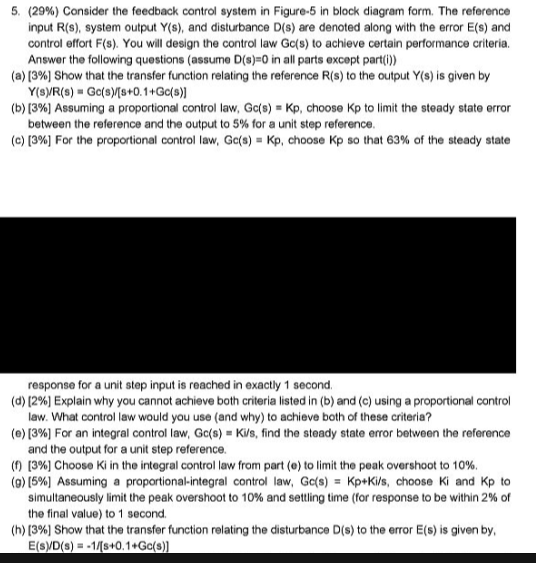5, (29%) Consider the feedback control system in Figure-5 in block diagram form. The reference input R(s), system output Y(s), and disturbance D(s) are denoted along with the error E(s) and control effort F(s). You will design the control law Gc(s) to achieve certain performance criteria. Answer the following questions (assume D(s)0 in all parts except part(ü) (a)  Show that the transfer function relating the reference R(s) to the output Y(s) is given by (b) [3%) Assuming a proportional...

• Find the transfer function for the given block diagram as shown in figure below by using...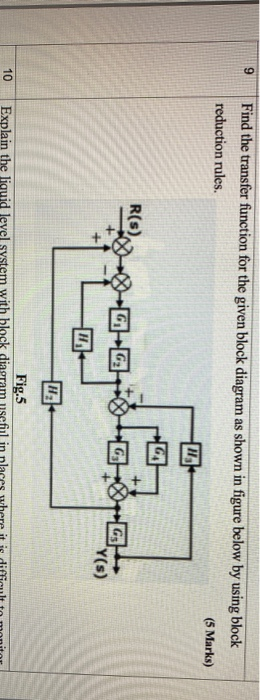Find the transfer function for the given block diagram as shown in figure below by using block reduction rules. (5 Marks) DG-600GBG 10 Fig.5 Explain the liquid level system with block diagram useful in nlaces where it is difficult to monitor